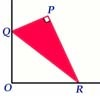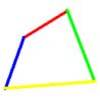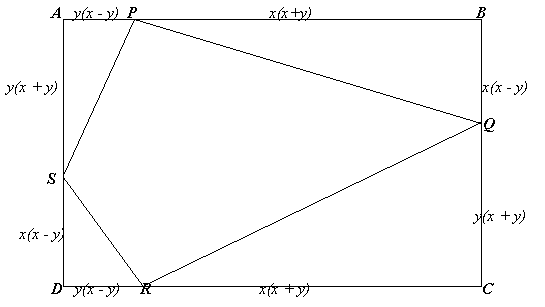You may also likeSet Square

A triangle PQR, right angled at P, slides on a horizontal floor with Q and R in contact with perpendicular walls. What is the locus of P?Biggest Bendy

Four rods are hinged at their ends to form a quadrilateral. How can you maximise its area?Strange Rectangle

ABCD is a rectangle and P, Q, R and S are moveable points on the edges dividing the edges in certain ratios. Strangely PQRS is always a cyclic quadrilateral and you can find the angles.

Strange Rectangle 2

Age 16 to 18Challenge LevelAs $x$ and $y$ change the points $S$, $P$, $Q$ and $R$ always lie on the circle with $SQ$ as diameter and the angles of the quadrilateral $SPQR$ remain the same. (See the problem Strange Rectangle from November 2001.)

Taking

$x = \sqrt{3}$ and $y = 1$

find all the angles in this figure and the exact values of the sine, cosine and tangent of these angles in surd form.

Now take

$x = \sqrt{2} + 1$ and $y = 1$

and find the exact values of the trigonometric ratios for $22.5^o$ and $67.5^o$